【一猫汽车网西安站】10万以内的热门小型SUV中，瑞虎3和瑞风S3一直是该级别的佼佼者，有着不错的销量和口碑。不久前新瑞虎3升级上市后竞争力进一步增强，对瑞风S3造成很大的冲击，如果这两款车在您的候选范围内，又不知道买哪款更划算，下面我们就对新瑞虎3和瑞风S3做一个全面的对比，让您有一个更加清晰的认知。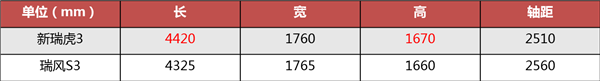外观方面正所谓萝卜白菜各有所爱，两款车也完全展现出不同的两个风格。从尺寸看，新瑞虎3的车身长度更长，瑞风S3的轴距更长，整体来说二者差距不大。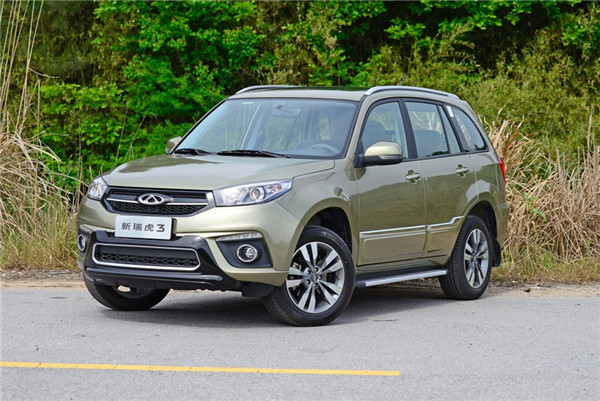新瑞虎3升级后外观变动不大，中网、车顶行李架、门把手等处进行了一些时尚化处理，依旧采用了“小书包”的外挂备胎方式，有点硬派越野的感觉。值得一提的是升级后新瑞虎3全系都采用了17寸铝合金轮毂，并标配车顶行李架，即便是入门车型也保证了视觉效果，顶配还增添了前保险杠护杠和侧踏板。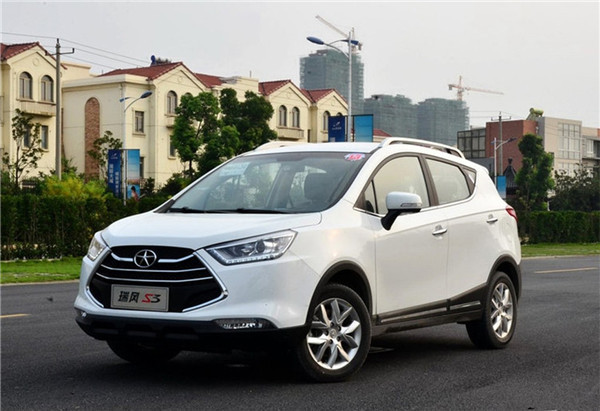瑞风S3标志性的大嘴中网非常有张力，识别度高，整车线条流畅，属于典型的都市SUV风格。不过瑞风S3中高配装备的是16寸铝合金轮毂，低配只配备了15寸钢制轮毂，轮胎宽度只有185mm，也没有车顶行李架，低配的视觉效果明显不如新瑞虎3。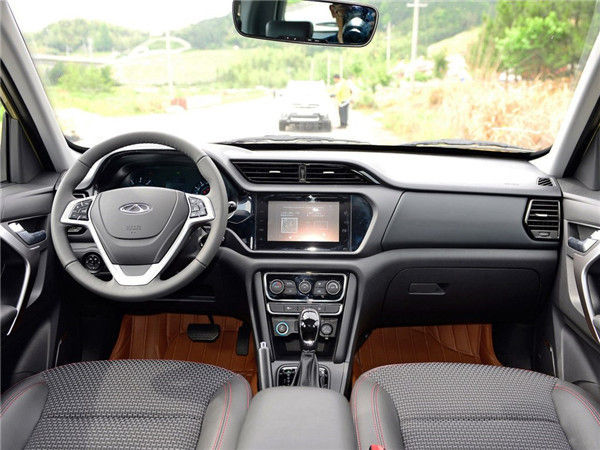升级后新瑞虎3最大的变化就是全新的内饰，采用不对称的设计，简约时尚。细节上，冰蓝自发光的仪表盘、粗壮运动的三幅方向盘、红线黑色的皮质拼接座椅，营造出动感的视觉效果。而且它的做工和用料的确比老款要好很多，整个内饰的质感有提升。

空间上新瑞虎3前后排头部空间充足，后排腿部空间够用但不充足，不过后备箱空间和整车储物空间表现却很出色，550L-1800L的后备箱空间达到同级车中最大容积，而且侧开尾门开口大，地板低，非常容易搬运大件物品。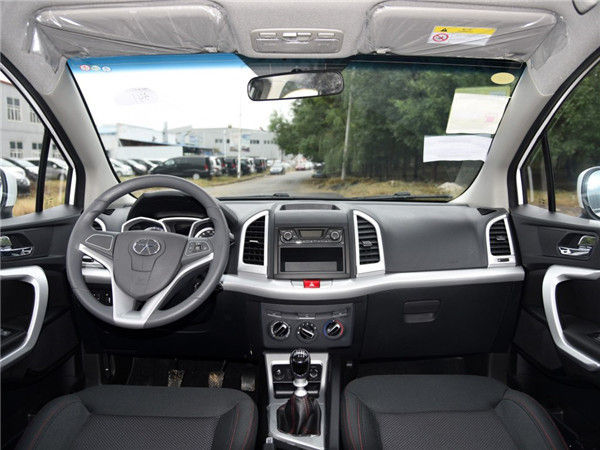相比之下瑞风S3的内饰就常规了很多，纯黑的内饰色调搭配一部分银色装饰，用来提升一些运动感，总的看来整体造型没有太多亮点，用料也中规中矩。

瑞风S3的乘坐空间表现突出，坐在后排腿部空间更宽松，头部空间也尚可，能够提供不错的乘坐舒适性。但储物空间没有新瑞虎3表现的好，455L-1254L的后备箱储物空间并不出众，在同级中也就是中游水平，而且车内储物格也没有新瑞虎3丰富。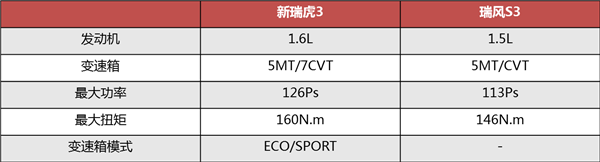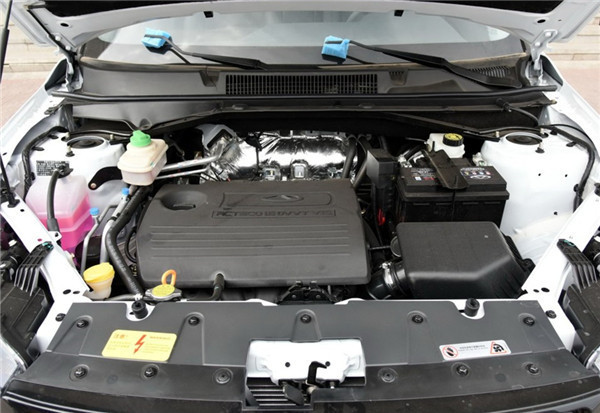新瑞虎3采用的是1.6L发动机，最大功率126马力，最大扭矩160N.n，配合手动变速箱或7CVT变速箱，动力在小型SUV中表现可圈可点。尤其是新瑞虎3的CVT变速箱拥有ECO（经济模式）和SPORT（运动模式），在动力性和燃油经济性上比瑞风S3更突出，可模拟7速的CVT变速箱也带来了更多的驾驶乐趣。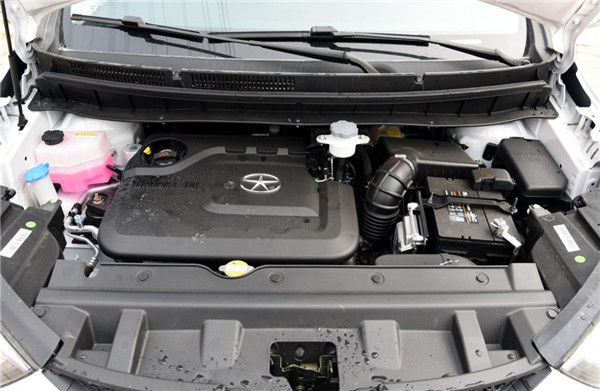瑞风S3搭载的是1.5L自然吸气发动机，最大功率113马力，最大扭矩146N.n，相比新瑞虎3动力输出明显弱一些，同样配备了手动变速箱和CVT变速箱。匹配电子助力系统，瑞风S3开起来非常轻松，超车时偶尔也需要大脚油门，超车嘛都要哄一下油门。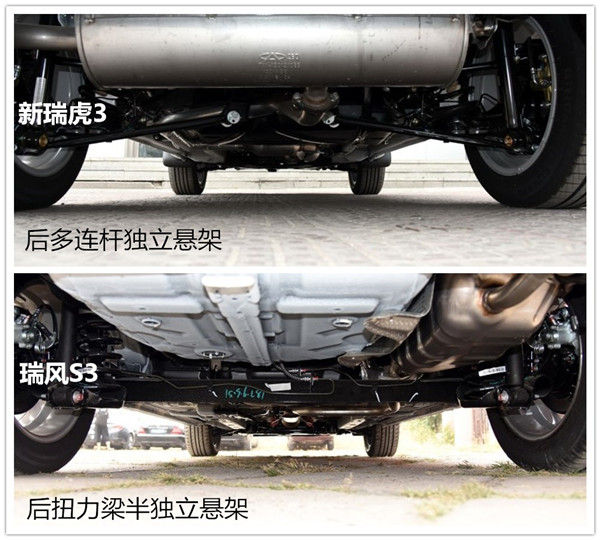底盘方面，新瑞虎3采用了前麦弗逊+后多连杆的四轮独立悬架结构，并且前后均配有横向稳定杆。瑞风S3则是前麦弗逊+后扭力梁半独立悬架，没有任何稳定杆。在舒适性上不如新瑞虎3，对于路面颠簸的过滤也更生硬，行驶质感没有新瑞虎3厚实，但是在平整路面瑞风S3是非常不错的。

配置是体现性价比的一个重要方面，两车配置上存在不小的差异。新瑞虎3 6.89万元的入门车型就配备了ESP电子车身稳定系统、牵引力控制、定速巡航、HHC坡道辅助、倒车雷达、多功能方向盘等配置，还是十分厚道的。相比之下6.58万元入门的瑞风S3配置相当寒酸，不仅这些均不具备，而且还采用了钢制轮圈和窄轮胎，甚至连后雨刷都省去了，一些最基础的安全配置不到位。

中高配车型中，新瑞虎3增加了自动空调、中控大屏、手机映射功能、胎压监控、行车记录仪和偏航预警等配置，而且可选装Cloudrive2.0智云娱乐行车系统，在安全、娱乐和科技配置上表现突出。而7.98万CVT豪华版的瑞风S3依然没有装备中控屏、还采用手动空调，只有顶配的车型的配置才相对完善，这方面被新上市的新瑞虎3甩开不少。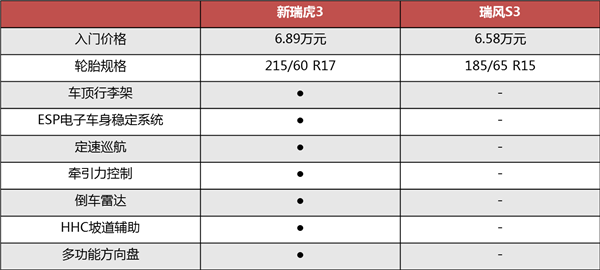通过对比不难看出，新瑞虎3升级后对瑞风S3确实造成了不小的威胁，主要体现在更全面的性能和非常完善的配置上。瑞风S3曾靠着时尚夸张的大嘴前脸和不错的车内空间赢得了一些市场，如今新瑞虎3强势归来，从整车均衡性能和性价比看，预算相同的前提下显然新瑞虎3更划算。

买车上一猫！

##### 相关资讯

|分享

•已阅！握爪
•我手滑为你点赞
•128个赞！
•不明觉厉
•阅后既醉
•有钱！任性
•照片太美，我不敢看
•干货！杠杠的
•高大上
•膜拜中！
•请接受我的膝盖
•猫哥/猫妹么么哒
•神吐槽
•我只笑笑不说话
•我想静静
•窒息
##### 资讯相关车系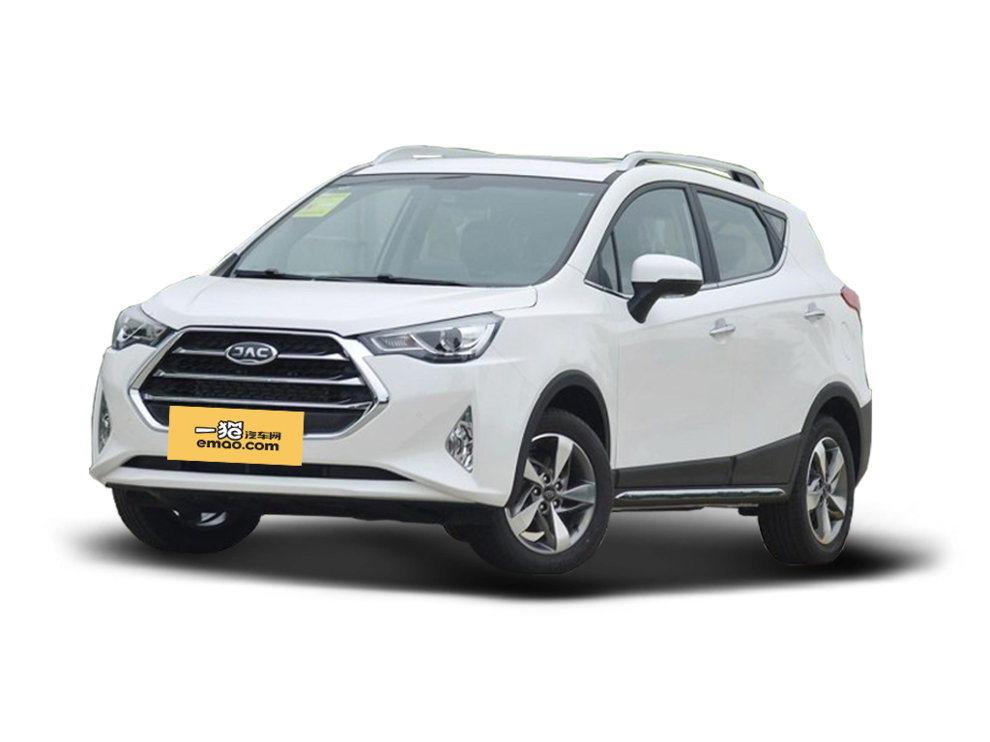##### 热度排行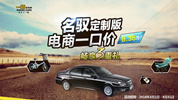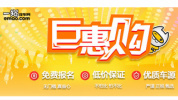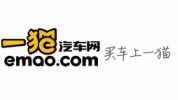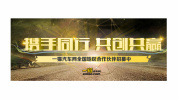• 阿斯顿·马丁
• 奥迪
• 阿尔法罗密欧
• ALPINA

• 巴博斯
• 宝骏
• 宝马
• 保时捷
• 北汽制造
• 奔驰
• 奔腾
• 本田
• 比亚迪
• 标致
• 别克
• 宾利
• 布加迪
• 北汽威旺
• 北京
• 北汽绅宝
• 北汽幻速
• 北汽新能源
• 宝沃
• 比速汽车
• 北汽道达

• 昌河
• 长安
• 长城
• 长安商用
• 成功汽车
• 长江EV
• 长安轻车型

• 大众
• 道奇
• 东风
• 东风风神
• 东风小康
• 东南
• DS
• 东风风行
• 东风风度
• 东风风光

• 法拉利
• 菲亚特
• 丰田
• 福迪
• 福特
• 福田汽车
• 福汽启腾
• 风诺

• GMC
• 广汽传祺
• 广汽吉奥
• 观致

• 哈飞
• 海格
• 海马
• 华泰
• 黄海
• 恒天
• 红旗
• 哈弗
• 华颂
• 华凯
• 华泰新能源
• 汉腾汽车

• Jeep
• 江淮
• 江铃
• 捷豹
• 金杯
• 九龙
• 吉利汽车
• 金旅
• 金龙
• 江铃集团轻汽
• 江铃集团新能源
• 君马汽车
• 捷途

• 开瑞
• 凯迪拉克
• 科尼赛克
• 克莱斯勒
• KTM
• 卡威
• 凯翼
• 康迪
• 康迪电动汽车集团

• 猎豹汽车
• 兰博基尼
• 劳斯莱斯
• 雷克萨斯
• 雷诺
• 理念
• 力帆
• 莲花汽车
• 林肯
• 铃木
• 陆风
• 路虎
• 路特斯
• 领志
• 领克
• 零跑汽车

• MG
• MINI
• 马自达
• 玛莎拉蒂
• 迈凯伦
• 摩根

• 纳智捷

• 讴歌
• 欧宝
• 欧朗
• 欧拉
• 欧尚汽车

• 帕加尼

• 奇瑞
• 启辰
• 起亚
• 前途
• 庆铃汽车

• 日产
• 荣威
• 瑞麒汽车
• 如虎
• 瑞驰

• 上汽大通
• smart
• 三菱
• 双环
• 双龙
• 斯巴鲁
• 斯柯达
• 萨博
• 思铭
• 赛麟
• SWM斯威汽车

• TESLA
• 泰卡特
• 腾势

• 威麟
• 威兹曼
• 沃尔沃
• 五菱汽车
• 五十铃
• 潍柴英致
• WEY
• 蔚来
• 威马汽车

• 现代
• 雪佛兰
• 雪铁龙
• 西雅特
• 新特汽车
• 小鹏汽车
• 新宝骏

• 野马汽车
• 一汽
• 依维柯
• 英菲尼迪
• 永源
• 驭胜

• 中华
• 中兴
• 众泰
• 知豆
• 之诺
• 正道汽车
• A
• B
• C
• D
• E
• F
• G
• H
• I
• J
• K
• L
• M
• N
• O
• P
• Q
• R
• S
• T
• U
• V
• W
• X
• Y
• Z

• 阿斯顿·马丁
• 奥迪
• 阿尔法罗密欧
• ALPINA

• 巴博斯
• 宝骏
• 宝马
• 保时捷
• 北汽制造
• 奔驰
• 奔腾
• 本田
• 比亚迪
• 标致
• 别克
• 宾利
• 布加迪
• 北汽威旺
• 北京
• 北汽绅宝
• 北汽幻速
• 北汽新能源
• 宝沃
• 比速汽车
• 北汽道达

• 昌河
• 长安
• 长城
• 长安商用
• 成功汽车
• 长江EV
• 长安轻车型

• 大众
• 道奇
• 东风
• 东风风神
• 东风小康
• 东南
• DS
• 东风风行
• 东风风度
• 东风风光

• 法拉利
• 菲亚特
• 丰田
• 福迪
• 福特
• 福田汽车
• 福汽启腾
• 风诺

• GMC
• 广汽传祺
• 广汽吉奥
• 观致

• 哈飞
• 海格
• 海马
• 华泰
• 黄海
• 恒天
• 红旗
• 哈弗
• 华颂
• 华凯
• 华泰新能源
• 汉腾汽车

• Jeep
• 江淮
• 江铃
• 捷豹
• 金杯
• 九龙
• 吉利汽车
• 金旅
• 金龙
• 江铃集团轻汽
• 江铃集团新能源
• 君马汽车
• 捷途

• 开瑞
• 凯迪拉克
• 科尼赛克
• 克莱斯勒
• KTM
• 卡威
• 凯翼
• 康迪
• 康迪电动汽车集团

• 猎豹汽车
• 兰博基尼
• 劳斯莱斯
• 雷克萨斯
• 雷诺
• 理念
• 力帆
• 莲花汽车
• 林肯
• 铃木
• 陆风
• 路虎
• 路特斯
• 领志
• 领克
• 零跑汽车

• MG
• MINI
• 马自达
• 玛莎拉蒂
• 迈凯伦
• 摩根

• 纳智捷

• 讴歌
• 欧宝
• 欧朗
• 欧拉
• 欧尚汽车

• 帕加尼

• 奇瑞
• 启辰
• 起亚
• 前途
• 庆铃汽车

• 日产
• 荣威
• 瑞麒汽车
• 如虎
• 瑞驰

• 上汽大通
• smart
• 三菱
• 双环
• 双龙
• 斯巴鲁
• 斯柯达
• 萨博
• 思铭
• 赛麟
• SWM斯威汽车

• TESLA
• 泰卡特
• 腾势

• 威麟
• 威兹曼
• 沃尔沃
• 五菱汽车
• 五十铃
• 潍柴英致
• WEY
• 蔚来
• 威马汽车

• 现代
• 雪佛兰
• 雪铁龙
• 西雅特
• 新特汽车
• 小鹏汽车
• 新宝骏

• 野马汽车
• 一汽
• 依维柯
• 英菲尼迪
• 永源
• 驭胜

• 中华
• 中兴
• 众泰
• 知豆
• 之诺
• 正道汽车
• A
• B
• C
• D
• E
• F
• G
• H
• I
• J
• K
• L
• M
• N
• O
• P
• Q
• R
• S
• T
• U
• V
• W
• X
• Y
• Z

• 阿斯顿·马丁
• 奥迪
• 阿尔法罗密欧
• ALPINA

• 巴博斯
• 宝骏
• 宝马
• 保时捷
• 北汽制造
• 奔驰
• 奔腾
• 本田
• 比亚迪
• 标致
• 别克
• 宾利
• 布加迪
• 北汽威旺
• 北京
• 北汽绅宝
• 北汽幻速
• 北汽新能源
• 宝沃
• 比速汽车
• 北汽道达

• 昌河
• 长安
• 长城
• 长安商用
• 成功汽车
• 长江EV
• 长安轻车型

• 大众
• 道奇
• 东风
• 东风风神
• 东风小康
• 东南
• DS
• 东风风行
• 东风风度
• 东风风光

• 法拉利
• 菲亚特
• 丰田
• 福迪
• 福特
• 福田汽车
• 福汽启腾
• 风诺

• GMC
• 广汽传祺
• 广汽吉奥
• 观致

• 哈飞
• 海格
• 海马
• 华泰
• 黄海
• 恒天
• 红旗
• 哈弗
• 华颂
• 华凯
• 华泰新能源
• 汉腾汽车

• Jeep
• 江淮
• 江铃
• 捷豹
• 金杯
• 九龙
• 吉利汽车
• 金旅
• 金龙
• 江铃集团轻汽
• 江铃集团新能源
• 君马汽车
• 捷途

• 开瑞
• 凯迪拉克
• 科尼赛克
• 克莱斯勒
• KTM
• 卡威
• 凯翼
• 康迪
• 康迪电动汽车集团

• 猎豹汽车
• 兰博基尼
• 劳斯莱斯
• 雷克萨斯
• 雷诺
• 理念
• 力帆
• 莲花汽车
• 林肯
• 铃木
• 陆风
• 路虎
• 路特斯
• 领志
• 领克
• 零跑汽车

• MG
• MINI
• 马自达
• 玛莎拉蒂
• 迈凯伦
• 摩根

• 纳智捷

• 讴歌
• 欧宝
• 欧朗
• 欧拉
• 欧尚汽车

• 帕加尼

• 奇瑞
• 启辰
• 起亚
• 前途
• 庆铃汽车

• 日产
• 荣威
• 瑞麒汽车
• 如虎
• 瑞驰

• 上汽大通
• smart
• 三菱
• 双环
• 双龙
• 斯巴鲁
• 斯柯达
• 萨博
• 思铭
• 赛麟
• SWM斯威汽车

• TESLA
• 泰卡特
• 腾势

• 威麟
• 威兹曼
• 沃尔沃
• 五菱汽车
• 五十铃
• 潍柴英致
• WEY
• 蔚来
• 威马汽车

• 现代
• 雪佛兰
• 雪铁龙
• 西雅特
• 新特汽车
• 小鹏汽车
• 新宝骏

• 野马汽车
• 一汽
• 依维柯
• 英菲尼迪
• 永源
• 驭胜

• 中华
• 中兴
• 众泰
• 知豆
• 之诺
• 正道汽车
• A
• B
• C
• D
• E
• F
• G
• H
• I
• J
• K
• L
• M
• N
• O
• P
• Q
• R
• S
• T
• U
• V
• W
• X
• Y
• Z